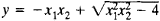# Entire Algebraic Function

The following article is from The Great Soviet Encyclopedia (1979). It might be outdated or ideologically biased.

## Entire Algebraic Function

An entire algebraic function of n variables is a function that satisfies an equation of the form

yk + p1yk–1 + · · · + pk = 0

where p1, . . ., pk are polynomials in n variables. For example,is an entire algebraic function, since y2 + 2x1x2y + 4 = 0.